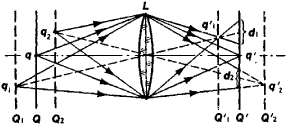# Depth of Image Space

The following article is from The Great Soviet Encyclopedia (1979). It might be outdated or ideologically biased.

## Depth of Image Space

the greatest distance, measured along the optical axis, between points in space that are depicted with adequate sharpness by an optical system (see Figure 1).Figure 1. Image produced by a lens L of the points in space lying in planes located at various distances from the lens: Q is the sighting plane, and Q′ is the focal plane. The point q is reflected sharply in the plane Q′, whereas the points q1 and q2 are reflected sharply in the planes Q′1 and Q′2. In the focal plane Q′, the points q1, and q2 are represented by blur circles of diameters d1, and d2, respectively.

The optical system forms a sharp image in the focal plane Q′ of only the points of a flat object that is perpendicular to the optical axis and situated at a fixed distance from the system (in the sighting plane Q). The points in the space that are situated in front of and behind the plane Q, in the planes Q1 and Q2, will be depicted sharply in the conjugate planes Q′1 and Q′2. In the focal plane Q1 these points will be represented by circles (blur circles) of finite dimensions d1 and d2, but if the diameter of the blur circles is less than a certain value (less than 0.1 mm for the normal eye), then the eye perceives them as points—that is, with equal sharpness. The distance between the planes Q1 and Q2 for which the corresponding points in a flat image or on a photograph appear to be equally sharp is called the depth of image space. The distance between the planes Q′1 and Q′2 is called the depth of focus (the distance Q1Q2 is also sometimes called the depth of focus).

The depth of image space varies inversely as the diameter of the entrance pupil of the lens. For this reason, in photographing an object with a foreground and background—that is, an object that extends along the optical axis of the system—the diaphragm aperture of the lens must be decreased.

### REFERENCE

Tudorovskii, A. I. Teoriia opticheskikh priborov. Moscow-Leningrad, 1952.

V. I. MALYSHEV

The Great Soviet Encyclopedia, 3rd Edition (1970-1979). © 2010 The Gale Group, Inc. All rights reserved.
Site: Follow: Share:
Open / Close